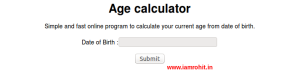# Create time range function in php

In this tutorial we’ll learn how to create a simple time range function with exceptional handling.
If you are looking for a solution to create time picker dropdown then there is already many solutions on internet, you can use jquery ui timepicker plugin, But if you don’t want to use extra plugin and want to make your code optimized and fast must try this function for generating time range in php.

Using PHP you can easily create a time range array that helps you to generate a quick time picker drop-down in HTML. In this short tutorial, I’ll show you how to create time range in PHP and HTML and display a time picker select dropdown using simple php time range function.Below I am going to create a php function so that you can add this time range function in your php library and call it where you want to add time range dropdown in your php project.

```<?php   function createTimeRange(\$startTime, \$endTime, \$interval = '15 mins', \$format = '12') { try {   if(!isset(\$startTime) || !isset(\$endTime)) { throw new exception("Start or End time is missing"); }   \$startTime = strtotime(\$startTime); \$endTime = strtotime(\$endTime);   \$currentTime = time(); \$addTime = strtotime('+'.\$interval, \$currentTime); \$timeDiff = \$addTime - \$currentTime;   \$timesArr = array(); \$timeFormat = (\$format == '12')?'g:i:s A':'G:i:s'; while (\$startTime < \$endTime) { \$timesArr[] = date(\$timeFormat, \$startTime); \$startTime += \$timeDiff; } return \$timesArr; } catch (Exception \$e) { return \$e->getMessage(); } }   ?>```

In the above function you have to pass two mandatory parameter Start and End time and can also pass two optional parameter as per your need.

\$startTime » Required. Specifies the start time. E.g., 7:30am or 7:30
\$endTime » Required. Specifies the end time. E.g., 8:15pm or 20:30
\$interval » Optional. Specifies the interval used in time range. E.g., 1 hour, 1 mins, 1 secs, etc
\$format » Optional. Specifies which time format you want to get in the time range. E.g., 12 or 24

Here is the example

`var_dump(createTimeRange("7:15", "11:30", "30min", 24));`

OutPut:

```array(9) { => string(7) "7:15:00" => string(7) "7:45:00" => string(7) "8:15:00" => string(7) "8:45:00" => string(7) "9:15:00" => string(7) "9:45:00" => string(8) "10:15:00" => string(8) "10:45:00" => string(8) "11:15:00" }```

Posted in PHP